# Practical exercise 3: THE NEIGHBOR ALSO LIKES IT

The mean distance between the Earth and the Moon is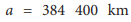and the mass of the Earth is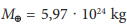.You can find out the period of the Moon's orbit from Task 2. Determine the mass of the Moon. How many times is the mass of the Earth greater than the mass of the Moon?

Solution:

We calculate the mass of the Moon from the exact 3rd Kepler´s law: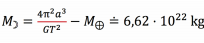.

For the orbital period, we substituted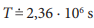, Because the mass of the Earth is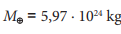, the Earth is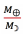90− times more massive than the Moon. The actual mass of the Moon is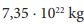, i.e.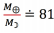, so the calculated result gives a reasonable estimate of the actual value.

Note that the calculated value of the mass of the Moon depends very much on the accuracy of the other quantities. If we substitute into the relation 27,3 ∙ 24 ∙ 3 600, as the orbital period, i.e. we did wouldn´t round it, we would get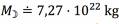, which obviously gives a more accurate result. However, this is a "coincidence", the correct procedure is to convert the orbital period from days to seconds and give the result as a number with the same number of digits as the default value, times the relevant power of ten, i.e. 27,3 dní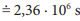.

Note: More precisely, it would be said that the result should have the same number of "valid digits" as the default value. The term "valid digit" is not even introduced in secondary school, so we tried to avoid this term.